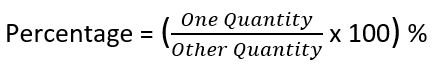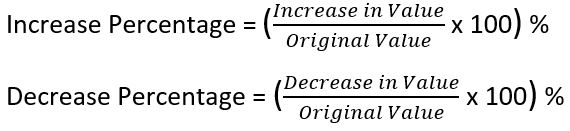## LetsPlayMaths.Com

WELCOME TO THE WORLD OF MATHEMATICS

# Class 7 Percentage

Introduction to Percentage

Conversion of Percentage into a Fraction

Conversion of Fraction into a Percentage

Conversion of Percentage into Ratio

Conversion of Ratio into Percentage

Conversion of Percentage into Decimal

Conversion of Decimal into Percentage

How to Find Percentage of a Given Quantity

Expressing One Quantity as Percentage of Another Quantity

Finding Increasing or Decreasing of Percentage

Use of Percentage in Our Life

Percentage Test

Percentage Worksheet

## Introduction to Percentage

The word percent means, per hundred or out of hundred. In other word Percentage is the numerator of a fraction with denominator 100. The symbol of percent is %. Let's see some examples where we use percentage in our day-to-day life.

Example 1. John got 85 marks out of hundred marks, then we can say he got 85 percent marks. In fraction form we can write it as 85100, here 85 is the mark secured and 100 is the total marks.

Example 2. 30 out of 50 students are girls in a school, then the percentage of girls in the school is 3050 = 30×250×2 = 60100 = 60%.

## Conversion of Percentage into a Fraction

To convert percentage into fraction, we must replace the % sign with 1100. Finally reduce the fraction to it's simplest form. Let's see some examples.

Examples 1. Express 20% in fraction form.

Solution. 20% = 20100 = 15

Example 2. Express 8.5% in fraction form.

Solution. 8.5% = 8.5100 = 851000 = 17200

## Conversion of Fraction into a Percentage

To convert a fraction into a percentage, we must multiply the fraction by 100 and write a % sign. Let's see some examples.

Example 1. Convert 25 into fraction.

Solution. 25 = 25 × 100 = 40%

Example 2. Convert 23 into fraction.

Solution. 23 × 100 = 2003 = 66 23%

## Conversion of Percentage into Ratio

To convert a percentage into a ratio, first we must convert into fraction to it's simplest form and then to a ratio. Let's see some examples.

Examples 1. Express 30% in fraction form.

Solution. 30% = 30100 = 310 = 3 : 10

Examples 2. Express 32.5% in fraction form.

Solution. 32.5% = 32.5100 = 3251000 = 1340 = 13 : 40

Examples 3. Express 22 25% in fraction form.

Solution. 22 25% = 1125 ÷ 100 = 1125 × 1100 = 28125 = 28 : 125

## Conversion of Ratio into Percentage

To convert a ratio into percentage, first we have to convert the given ratio into a fraction and then convert into a percentage. Let's see some examples.

Examples 1. Express 2 : 5 in percentage form.

Solution. 2 : 5 = 25 = 25 × 100 = 40%

Examples 2. Express 5 : 8 in percentage form.

Solution. 5 : 8 = 58 = 58 × 100 = 0.625 × 100 = 62.5%

## Conversion of Percentage into Decimal

To convert a percentage into a decimal, first convert the percentage into a fraction and then convert fraction into decimal. Let's see some examples.

Example 1. Convert 72% into decimal.

Solution. 72% = 72100 = 0.72

Example 2. Convert 175% into decimal.

Solution. 175% = 175100 = 1.75

Example 3. Convert 38.5% into decimal.

Solution. 38.5% = 38.5100 = 0.385

## Conversion of Decimal into Percentage

To convert a decimal into a percentage, we have to multiply the decimal by 100 and put % sign. Let's see some examples.

Example 1. Convert 0.65 into percentage.

Solution. 0.65 = 0.65 × 100 = 65%

Example 2. Convert 2.75 into percentage.

Solution. 2.75 = 2.75 × 100 = 275%

## How to Find Percentage of a Given Quantity

To find a percentage of a given quantity, we must change the percentage into fraction and then multiply it by the given quantity. Let's see some examples.

Example 1. What is the value of 25% of 60?

Solution. 25% of 60 = 25100 × 60 = 604 = 15

Example 2. Find the value of 15% of Rs. 80.

Solution. 15% of Rs. 80 = 15100 × 80 = 320 × 80 = 3 × 4 = Rs. 12

## Expressing One Quantity as Percentage of Another Quantity

To express one quantity as a percentage of another same quantity, we must divide one quantity with the other quantity and then multiply the result with 100.Let's see some examples.

Example 1. Express 25 as a percentage of 75.

Solution. Percentage = (2575 × 100)%

= 33 13 %

Example 2. Express 500 grams as percentage of 2.5 kg.

Solution. 2.5 kg = 2500 grams

Percentage = 5002500 × 100 = 20%

## Finding Increasing or Decreasing of PercentageLet's see some examples to understand this.

Example 1. A cycle was costing Rs. 4500 last year, but this year it is costing Rs. 5000. Find the percentage increase in the price.

Solution. Original price = Rs. 4500

Rise in price = Rs. 5000 − Rs. 4500 = Rs. 500

Percentage increase = 5004500 × 100 = 1009 = 11 19%

Hence, the price has increased by 11 19%

Example 2. Price of orange per kilogram is Rs. 60 last year. This year orange price is Rs. 50 pr kilogram. Find the percentage decrease in price.

Solution. Original price = Rs. 60

Fall in price = Rs. 60 − Rs. 50 = Rs. 10

Percentage decrease = 1060 × 100 = 1006 = 503 = 16 23%

Hence, the price has decreased by 16 23%

## Use of Percentage in Our Life

Let's see some real life problems on percentage.

Example 1. In a school of 200 students, 45% students are boys. How many girls are present in the school?

Solution. Boys percentage in the school is 45%.

Girls percentage in the school = 100 − 45 = 55%

Number of girls in the school = 55% of 200 = 55100 × 200 = 110

So, the number of girls present in the school is 110.

Example 2. Population of a country is 750000. It is forecasted that the population of the country will rise by 6% after 10 years. What will be the population of country after 10 years.

Solution. Population of the country = 750000

Rise in population = 6% of 750000

= 6100 × 750000 = 45000

Total population after 10 years = 750000 + 45000 = 795000

So, the population of the country after 10 years will be 795000.

Example 3. 25% of a number is 75, then find the number.

Solution. Let's assume the number is 'a'.

25% of a = 75

=> 25100 × a = 75

=> a = 75 × 10025

=> a = 300

So, the number is 300.

Example 4. John bought a motor bike at a discount of 25% and he saved 15000. What was the price of the motorbike before discount?

Solution. Let's assume original price of the motorbike is 'q'.

q − 25% of q = 15000

=> q − 25q100 = 15000

=> 100q−25q100 = 15000

=> 75q100 = 15000

=> q = 15000 × 10075

=> q = 20000

So, the original price of the motorbike is Rs. 20000

## Class-7 Percentage Test

Percentage Test - 1

Percentage Test - 2

## Class-7 Percentage Worksheet

Percentage Worksheet - 1

Percentage Worksheet - 2

Percentage Worksheet - 3